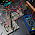# How to Save Float Data To Arduino EEPROM

Saving Float Data To EEPROM - Float is a comma numeric data and this float value is widely used for values that have commas such as coordinates, analog voltages, and measurements with high accuracy.

This float value is stored in memory with a capacity of 4 bytes or 32 bits. The range of stored values is 3.4028235E+38 to -3.4028235E+38.

Not only float, if you want to store with a larger value, you can use double.

## How to Save Float Value To EEPROM

If we usually save integer values or integers to the EEPROM using the "write" command, it's different if we want to store comma numbers. the command used is "put". So the program line is:

The destination address is not static, meaning that the address is only given from where the data starts to be stored and will increase with the amount of data.

For example if using the command "EEPROM.write", if the value you want to store is an integer "5" and will be stored at the address to "1".

Then the storage memory for the number "5" only exists at the address to "1". Unlike "EEPROM.put", if we store the value "5.12345" to address "1", then the memory used starts from:

• the address "first" stores the number 5,
• the address "second" stores the "."
• the address "third" stores the number 2
• and so on.

Well, if the data to be stored is two data such as "float data1 = 1.2321;" and "float data2 = 34,324;", then write the address as follows:
EEPROM.put(1, data1);
EEPROM.put(7, data2);

## Write and Read Float Value EEPROM

Read the float value from the EEPROM using the "get" command with an example program line:

```#include <EEPROM.h>

float a = 96.99419; //Data yang akan di simpan
float b;

void setup() {
Serial.begin(9600);

//Simpan data float ke EEPROM
Serial.print("Tulis ke EEPROM : ");
Serial.println(a, 5);
delay(1000);

//Ambil data float ke EEPROM
Serial.print("Baca dari EEPROM : ");
Serial.println(b, 5);
delay(1000);
}

void loop()
{
//Kosong
}
```

Results is:

## 2 comments for "How to Save Float Data To Arduino EEPROM"

1.1.Sama-sama.